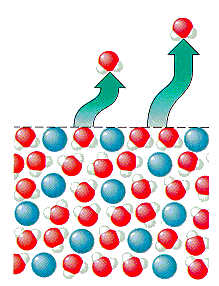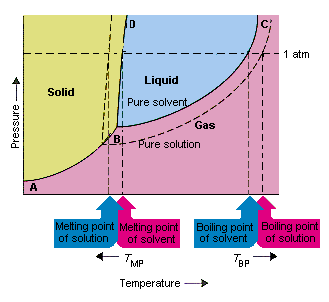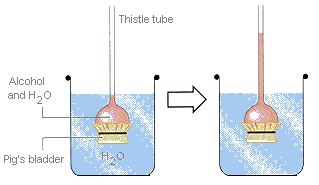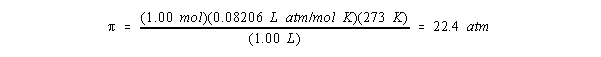Colligative Properties

Colligative Properties

 Vapor Pressure Depression Boiling Point Elevation and Freezing Point Depression Colligative Properties Calculations Osmotic Pressure

Vapor Pressure Depression

Physical properties can be divided into two categories. Extensive properties (such as mass and volume) depend on the size of the sample. Intensive properties (such as density and concentration) are characteristic properties of the substance; they do not depend on the size of the sample being studied. This section introduces a third category that is a subset of the intensive properties of a system. This third category, known as colligative properties, can only be applied to solutions. By definition, one of the properties of a solution is a colligative property if it depends only on the ratio of the number of particles of solute and solvent in the solution, not the identity of the solute.

Very few of the physical properties of a solution are colligative properties. As an example of this limited set of physical properties, let's consider what happens to the vapor pressure of the solvent when we add a solute to form a solution. We'll define Po as the vapor pressure of the pure liquidthe solventand P as the vapor pressure of the solvent after a solute has been added.

Po = vapor pressure of the pure liquid, or solvent

P = vapor pressure of the solvent in a solution

When the temperature of a liquid is below its boiling point, we can assume that the only molecules that can escape from the liquid to form a gas are those that lie near the surface of the liquid.

When a solute is added to the solvent, some of the solute molecules occupy the space near the surface of the liquid, as shown in the figure below. When a solute is dissolved in a solvent, the number of solvent molecules near the surface decreases, and the vapor pressure of the solvent decreases.This has no effect on the rate at which solvent molecules in the gas phase condense to form a liquid. But it decreases the rate at which the solvent molecules in the liquid can escape into the gas phase. As a result, the vapor pressure of the solvent escaping from a solution should be smaller than the vapor pressure of the pure solvent.

 P < Po vapor pressure of the solvent above a solution vapor pressure of the pure solvent

Between 1887 and 1888, Francois-Marie Raoult showed that the vapor pressure of a solution is equal to the mole fraction of the solvent times the vapor pressure of the pure liquid.

 P = Csolvent Po vapor pressure of the solvent above a solution vapor pressure of the pure solvent

This equation, which is known as Raoult's law, is easy to understand. When the solvent is pure, and the mole fraction of the solvent is equal to 1, P is equal to Po. As the mole fraction of the solvent becomes smaller, the vapor pressure of the solvent escaping from the solution also becomes smaller.

Let's assume, for the moment, that the solvent is the only component of the solution that is volatile enough to have a measurable vapor pressure. If this is true, the vapor pressure of the solution will be equal to the vapor pressure of the solvent escaping from the solution. Raoult's law suggests that the difference between the vapor pressure of the pure solvent and the solution increases as the mole fraction of the solvent decreases.

The change in the vapor pressure that occurs when a solute is added to a solvent is therefore a colligative property. If it depends on the mole fraction of the solute, then it must depend on the ratio of the number of particles of solute to solvent in the solution but not the identity of the solute.Boiling Point Elevation and Freezing Point Depression

The figure below shows the consequences of the fact that solutes lower the vapor pressure of a solvent. The solid line connecting points B and C in this phase diagram contains the combinations of temperature and pressure at which the pure solvent and its vapor are in equilibrium. Each point on this line therefore describes the vapor pressure of the pure solvent at that temperature. The dotted line in this figure describes the properties of a solution obtained by dissolving a solute in the solvent. At any given temperature, the vapor pressure of the solvent escaping from the solution is smaller than the vapor pressure of the pure solvent. The dotted line therefore lies below the solid line.The decrease in the vapor pressure of the solvent that occurs when a solute is added to the solvent causes an increase in the boiling point and decrease in the melting point of the solution.

According to this figure, the solution can't boil at the same temperature as the pure solvent. If the vapor pressure of the solvent escaping from the solution is smaller than the vapor pressure of the pure solvent at any given temperature, the solution must be heated to a higher temperature before it boils. The lowering of the vapor pressure of the solvent that occurs when it is used to form a solution therefore increases the boiling point of the liquid.

When phase diagrams were introduced, the triple point was defined as the only combination of temperature and pressure at which the gas, liquid, and solid can exist at the same time. The figure above shows that the triple point of the solution occurs at a lower temperature than the triple point of the pure solvent. By itself, the change in the triple point is not important. But it results in a change in the temperature at which the solution freezes or melts. To understand why, we have to look carefully at the line that separates the solid and liquid regions in the phase diagram. This line is almost vertical because the melting point of a substance is not very sensitive to pressure.

Adding a solute to a solvent doesn't change the way the melting point depends on pressure. The line that separates the solid and liquid regions of the solution is therefore parallel to the line that serves the same function for the pure solvent. This line must pass through the triple point for the solution, however. The decrease in the triple point that occurs when a solute is dissolved in a solvent therefore decreases the melting point of the solution.

The figure above shows how the change in vapor pressure that occurs when a solute dissolves in a solvent leads to changes in the melting point and the boiling point of the solvent as well. Because the change in vapor pressure is a colligative property, which depends only on the relative number of solute and solvent particles, the changes in the boiling point and the melting point of the solvent are also colligative properties.Colligative Properties Calculations

The best way to demonstrate the importance of colligative properties is to examine the consequences of Raoult's law. Raoult found that the vapor pressure of the solvent escaping from a solution is proportional to the mole fraction of the solvent.

P = Csolvent Po

But the vapor pressure of a solvent is not a colligative property. Only the change in the vapor pressure that occurs when a solute is added to the solvent can be included among the colligative properties of a solution.

Because pressure is a state function, the change in the vapor pressure of the solvent that occurs when a solute is added to the solvent can be defined as the difference between the vapor pressure of the pure solvent and the vapor pressure of the solvent escaping from the solution.

P = Po - P

Substituting Raoult's law into this equation gives the following result.

P = Po - Csolvent Po = (1 - Csolvent) Po

This equation can be simplified by remembering the relationship between the mole fraction of the solute and the mole fraction of the solvent.

Csolute + Csolvent = 1

Substituting this relationship into the equation that defines P gives another form of Raoult's law.

P = Csolute Po

This equation reminds us that the change in the vapor pressure of the solvent that occurs when a solute is added to the solvent is proportional to the mole fraction of the solute. As more solute is dissolved in the solvent, the vapor pressure of the solvent decreases, and the change in the vapor pressure of the solvent increases.

Because changes in the boiling point of the solvent (TBP) that occur when a solute is added to a solvent result from changes in the vapor pressure of the solvent, the magnitude of the change in the boiling point is also proportional to the mole fraction of the solute.TBP = kb solute

In dilute solutions, the mole fraction of the solute is proportional to the molality of the solution, as shown in the figure below.The equation that describes the magnitude of the boiling point elevation that occurs when a solute is added to a solvent is therefore often written as follows.TBP = kbm

Here,TBP is the boiling point elevation -- the change in the boiling point that occurs when a solute dissolves in the solventand kb is a proportionality constant known as the molal boiling point elevation constant for the solvent.

A similar equation can be written to describe what happens to the freezing point (or melting point) of a solvent when a solute is added to the solvent.TFP = -kf m

In this equation,TFP is the freezing point depressionthe change in the freezing point that occurs when the solute dissolves in the solvent -- and kf is the molal freezing point depression constant for the solvent. A negative sign is used in this equation to indicate that the freezing point of the solvent decreases when a solute is added.

Values of kf and kb as well as the freezing points and boiling points for a number of pure solvents are given in the tables below.

Freezing Point Depression Constants

 Compound Freezing Point (oC) kf (oC/m) water 0 1.853 acetic acid 16.66 3.90 benzene 5.53 5.12 p-xylene 13.26 4.3 naphthalene 80.29 6.94 cyclohexane 6.54 20.0 carbon tetrachloride -22.95 29.8 camphor 178.75 37.7

Boiling Point Elevation Constants

 Compound Boiling Point (oC) kb (oC/m) water 100 0.515 ethyl ether 34.55 1.824 carbon disulfide 46.23 2.35 benzene 80.10 2.53 carbon tetrachloride 76.75 4.48 camphor 207.42 5.611

 Practice Problem 6:Calculate the molecular weight of sulfur if 35.5 grams of sulfur dissolve in 100.0 grams of CS2 to produce a solution that has a boiling point of 49.48oC. Click here to check your answer to Practice Problem 6 Click here to see a solution to Practice Problem 6

 Practice Problem 7:Determine the molecular weight of acetic acid if a solution that contains 30.0 grams of acetic acid per kilogram of water freezes at -0.93oC. Do these results agree with the assumption that acetic acid has the formula CH3CO2H? Click here to check your answer to Practice Problem 7 Click here to see a solution to Practice Problem 7

What would happen in the calculation in Practice Problem 7 were repeated with a stronger acid, such as hydrochloric acid?

 Practice Problem 8:Explain why an 0.100 m solution of HCl dissolved in benzene has a freezing point depression of 0.512oC, while an 0.100 m solution of HCl in water has a freezing point depression of 0.352oC. Click here to check your answer to Practice Problem 8

In 1884 Jacobus Henricus van't Hoff introduced another term into the freezing point depression and boiling point elevation expressions to explain the colligative properties of solutions of compounds that dissociate when they dissolve in water.TFP = -kf (i) m

Substituting the experimental value for the freezing point depression of an 0.100 m HCl solution into this equation gives a value for the i term of 1.89. If HCl did not dissociate in water, i would be 1. If it dissociates completely, i would be 2. The experimental value of 1.89 suggests at least 95% of the HCl molecules dissociate in this solution.

 Practice Problem 9:Explain why 0.60 grams of acetic acid dissolve in 200 grams of benzene to form a solution that lowers the freezing point of benzene to 5.40oC. Click here to check your answer to Practice Problem 9Osmotic Pressure

In 1784, the French physicist and clergyman Jean Antoine Nollet discovered that a pig's bladder filled with a concentrated solution of alcohol in water expanded when it was immersed in water. The bladder acted as a semipermeable membrane, which allowed water molecules to enter the solution, but kept alcohol molecules from moving in the other direction. Movement of one component of a solution through a membrane to dilute the solution is called osmosis, and the pressure this produces is called the osmotic pressure ().

Osmotic pressure can be demonstrated with the apparatus shown in the figure below. A semipermeable membrane is tied across the open end of a thistle tube. The tube is then partially filled with a solution of sugar or alcohol in water and immersed in a beaker of water. Water will flow into the tube until the pressure on the column of water due to the force of gravity balances the osmotic pressure driving water through the membrane.Water flows through the semipermeable membrane to dilute the alcohol solution until the force of gravity pulling down on the column of this solution balances the osmotic pressure pushing the water through the membrane.

The same year that Raoult discovered the relationship between the vapor pressure of a solution and the vapor pressure of a pure solvent, Jacobus Henricus van't Hoff found that the osmotic pressure of a dilute solution () obeyed an equation analogous to the ideal gas equation.= nRT V

This equation suggests that osmotic pressure is another example of a colligative property, because this pressure depends on the ratio of the number of solute particles to the volume of the solutionn/Vnot the identity of the solute particles. It also reminds us of the magnitude of osmotic pressure. According to this equation, a 1.00 M solution has an osmotic pressure of 22.4 atm at 0oC.This means that a 1.00 M solution should be able to support a column of water 670 inches, or almost 56 feet, tall!

Biologists and biochemists often take advantage of osmotic pressure when they isolate the components of a cell. When a cell is added to an aqueous solution that contains a much higher concentration of ions than the liquid within the cell, water leaves the cell by flowing through the cell membrane until the cell shrinks so much that the membrane breaks. Alternatively, when a cell is placed in a solution that has a much smaller ionic strength, water pours into the cell, and the cell expands until the cell membrane bursts.